SIFT算子

Posted by GwanSiu on May 27, 2017

1.Introduction

SIFT(Scale Invariant Feature Transform) featuers are invariant to image scaling and ratation, and patially robust to change in illumination and 3D camera viewpoint. SIFT descriptor is a classical local descriptor, which has been applied for many computer vision applications, such as image classification, image matching, and etc.

The properties of SIFT features:

1. SIFT features are invariant to image scaling and roration, and patially invariant to change in illumination and 3D camera viewpoint. The featues are also robust to illuminatin, occlusion and affine transform.
2. SIFT features are highly distinctive, which allows a single feature to be correctly matched with high probability against a large database of features, providing a basis for object and scene recognition.
3. Lots of numbers of features can be generaed from few objects.
4. Real-Time: For object recognition, SIFT features can be matched quickly in large database.
5. SIFT also have the the ability to detect small objects in cluttered backgrounds requires that at least 3 features be correctly matched from each object for reliable identification.

In this blog, we simply summary SIFT featues and its properties. In section 2, the produce of SIFT features caculation is introduced. In section 3, we will analysis SIFT features in depth and try to answer the question: why are SIFT features invariant to image scaling and rotation? why are SIFT featues robust to illumination? In addition, this blog is written in English and Chinese.

2.The Process of SIFT calculation(摘抄原文)

1. Scale-space extrema detection（尺度空间的极点检测）:The first stage of computation searches over all scales and image locations. It is implemented efficiently by using a difference-of-Gaussian function to identify potential interest points that are invariant to scale and orientation. （所有图像所有尺度和位置，使用difference-of-Gaussian function检测出具有尺度不变性和方向不变性的interst points）
2. Keypoint localization(特征点定位): At each candidate location, a detailed model is fit to determine location and scale. Keypoints are selected based on measures of their stability. (这一步是在所有的特征点中，选择出具有稳定性的特征点)
3. Orientation assignment(赋予方向): One or more orientations are assigned to each keypoint lo- cation based on local image gradient directions. All future operations are performed on image data that has been transformed relative to the assigned orientation, scale, and location for each feature, thereby providing invariance to these transformations.
4. Keypoint descriptor(特征点描述子): The local image gradients are measured at the selected scale in the region around each keypoint. These are transformed into a representation that allows for significant levels of local shape distortion and change in illumination.

2.1 Scale-space extrema detection

1. Construct image scale space based on Gaussian function:

where, $\ast$ is the convolution operation in x and y, and

Using scale-space extrema in the difference-of-Guassian function convolved with the image to efficiently detect stable keypoint locations in the scale space.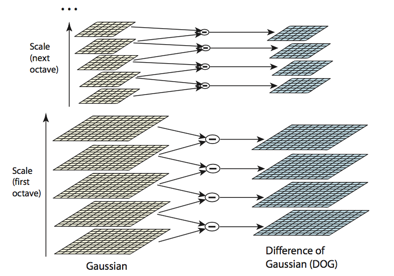One octave represents one resolution of an image. In each octave, different scale-space of the image are constructed by the gaussian function. Adjacent Gaussian images are subtracted to produce the difference-of-Gaussian images on the right. After each octave, the Gaussian image is down-sampled by a factor of 2, and the process repeated.

1. Why is gaussian function used to construct scale space?

Koenderink (1984) and Lindeberg (1994) has proof that Gasussian function is only one scale-space kernel in linear space, and thus to construct scale space by Gaussian function is reasonable.

2. Why is difference of gaussian function a scale invariant features detector?

Difference-of-Gaussian function approximates to the the scale-normalized gaussian, $\sigma^{2}\nabla^{2}G$. Lindeberg (1994) claimed in his research：scale-normalized Laplacian of Gaussian, $\sigma^{2}\nabla^{2}G$, with scale-invariant property，and the maximum and minimum of the $\sigma^{2}\nabla^{2}G$ can produce the most stable image features compared to a range of other possible image funcitons, such as gradient, Hessian, or Harris corner function.
The relationship between $D$ and $\sigma\nabla^{2}G$:

and $\begin{equation} \sigma \nabla^{2}G = \frac{\partial G}{\partial \sigma}\approx \frac{G(x,y,k\sigma)-G(x,y,\sigma)}{k\sigma - \sigma} \end{equation}$

Thus: $\begin{equation} G(x,y,k\sigma)-G(x,y,\sigma) \approx (k-1)\sigma^{2}\nabla^{2}G \end{equation}$

we can see that the difference between difference-of-gaussian function and scale-normalized gaussian function is scale factor of $\sigma^{2}$，where $(k-1)$ is constant and don’t effect the location of extrema.

1. local extrema detection how to locate local extrema（including local maxima and minima）?

Each sample point is compared to its eight neighbors in the current image and nine neighbors in the sccale above and below.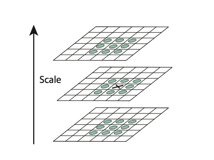2.2 Keypoint localization

2.2.1 Accurate keypoint localization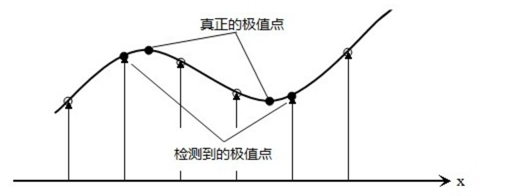Why is the function value at the extremumm, $D(\hat{x})$ useful for rejecting unstable extrema with low constrast. 未填

2.2.2 Eliminating Edge Responses

what’s the stability for the keypoint? 文中指出：for stability, it is not sufficient to reject keypoints with low constrast,The difference-of- Gaussian function will have a strong response along edges, even if the location along the edge is poorly determined and therefore unstable to small amounts of noise.

The priciple curvatures can be computed by the a $2x2$ Hessian matrix, $H$, is computed at the location and sccale of the keypoint:

which depends only on the ratio of the eigenvalues rather than their individual values. The quantity $(r + 1)^{2}/r$ is at a minimum when the two eigenvalues are equal and it increases with r. Therefore, to check that the ratio of principal curvatures is below some threshold, r, we only need to check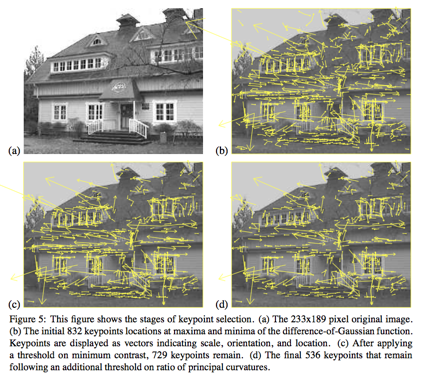2.3 Orientation assignment

For each Keypoint, it contains information of location$(x,y)$, scale, and orientation, and thus it’s scale-invariant, shift-invariant, and orientation-invariant.

2.4 Descriptor representation（梯度方向直方图，128维向量）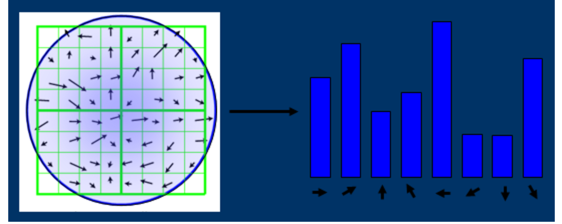1. 确定计算local discriptor生成的neighborhood, 可以是矩形，也可以是圆，这里以圆为例子:

where, $\sigma_{oct}$ is the scale function, $d=4$.

1. 将领域内的像素做affine transform, 旋转至关键点主方向.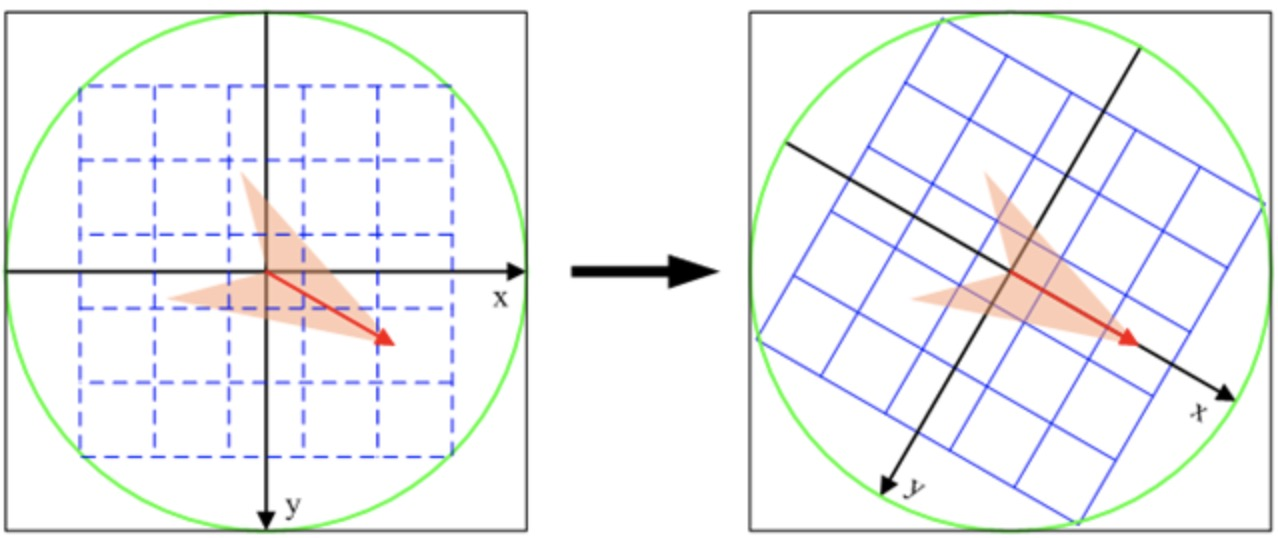1. 对图像领域内的像素求梯度方向以及幅值，对每个梯度幅值乘以高斯权重生成直方图。Gradient gistogram of local descriptor 是关键点所在尺度的模糊图像计算产生，生成直方图的计算如下：

where $x_{k}$是该点与关键点的列距离, $y_{k}$是该点与关键点的行距离，$\sigma_{\omega}$ 等于描述子窗口宽度$3\sigma$直方图列数取一半, $\omega$ 表示直方图某个bin的数值。

1. 在窗口宽度为$2\times 2$的区域内计算8个方向的梯度直方图，累计每个方向的数值，形成该区域内的梯度直方图。然后在下一个$2\times 2$区域重复该步骤。共生成16个梯度直方图。

2. Normalization:

Raw descriptor: $\omega=(\omega_{1},\omega_{2},…,\omega_{128})$ Normalized Feature: $I=(I_{1},I_{2},…,I_{128})$

Reference